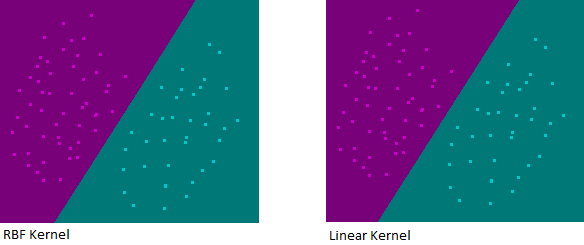# Linear Kernel: Why is it recommended for text classification ?

The Support Vector Machine can be viewed as a kernel machine. As a result, you can change its behavior by using a different kernel function.

The most popular kernel functions are :

• the linear kernel
• the polynomial kernel
• the RBF (Gaussian) kernel
• the string kernel

## The linear kernel is often recommended for text classification

It is interesting to note that :

The original optimal hyperplane algorithm proposed by Vapnik in 1963 was a linear classifier 

That's only 30 years later that the kernel trick was introduced.

If it is the simpler algorithm, why is the linear kernel recommended for text classification?

## Text is often linearly separable

Most of text classification problems are linearly separable 

## Text has a lot of features

The linear kernel is good when there is a lot of features. That's because mapping the data to a higher dimensional space does not really improve the performance.   In text classification, both the numbers of instances (document) and features (words) are large.As we can see in the image above, the decision boundary produced by a RBF kernel when the data is linearly separable is almost the same as the decision boundary produced by a linear kernel. Mapping data to a higher dimensional space using an RBF kernel was not useful.

## Linear kernel is faster

Training a SVM with a linear kernel is faster than with another kernel. Particularly when using a dedicated library such as LibLinear 

## Less parameters to optimize

When you train a SVM with a linear kernel, you only need to optimize the C regularization parameter.  When training with other kernels, you also need to optimize the $\gamma$ parameter which means that performing a grid search will usually take more time.

## Conclusion

Linear kernel is indeed very well suited for text-categorization.

Keep in mind however that it is not the only solution and in some case using another kernel might be better.

The recommended approach for text classification is to try a linear kernel first, because of its advantages.
If however you search to get the best possible classification performance, it might be interesting to try the other kernels to see if they help.